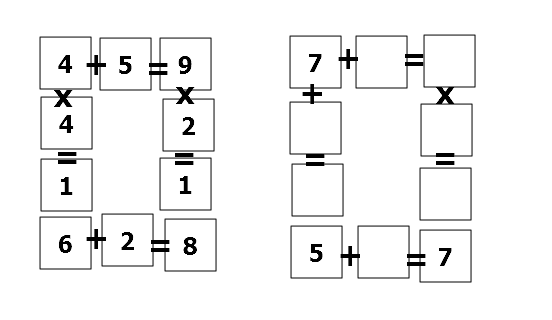# Millions of combinationsThe above shows two arithmetic puzzles: the left one is complete while the right one is incomplete.

Each box can only contain a non-zero digit.

Find the sum of all the missing digits in the puzzle on the right.

×